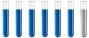## Normality of a solution

Chemistry and homework help forum.

Organic Chemistry, Analytical Chemistry, Biochemistry, Physical Chemistry, Computational Chemistry, Theoretical Chemistry, High School Chemistry, Colledge Chemistry and University Chemistry Forum.

Share your chemistry ideas, discuss chemical problems, ask for help with scientific chemistry questions, inspire others by your chemistry vision!

Please feel free to start a scientific chemistry discussion here!

Discuss chemistry homework problems with experts!

Ask for help with chemical questions and help others with your chemistry knowledge!

Moderators: expert, ChenBeier, Xen

Dhamnekar Winod
Sr. Staff MemberPosts: 198
Joined: Sat Nov 21, 2020 10:14 am
Location: Mumbai[Bombay],Maharashtra State,India

### Normality of a solution

My answer to the following question is 0.02 N But the answer given is 0.2N. Where I am wrong?

Question :Calculate the normality of NaOH solution formed by dissolving 0.2 gm NaOH to make 250 ml solution.

Solution:

Normality (N) = $$\frac{number of Gram Equivalent of solute}{Volume of Solution in litre}$$
Number of Gram Eq. of the Solute = $$\frac{weight}{Equivalent weight}$$
Now, Equivalent weight= Molar Mass n =23+16+11=40

So, N =$$\frac{ No.of gram eq.mass}{Vol (liter)}$$
$$= \frac{Weight}{Equivalent weight × 1000/V(in ml)}$$
= $$\frac{2}{40} \times \frac{1000}{250}$$
= 0.2 N

Last edited by Dhamnekar Winod on Sat Sep 04, 2021 8:06 pm, edited 1 time in total.
Any science consists of the following process. 1) See 2)Hear 3)Smell,if needed 4)Taste, if needed 5)Think 6)Understand 7)Inference 8)Take decision [Believe or disbelieve, useful or useless, true or false, cause or effect, any other criteria]
ChenBeier
Distinguished MemberPosts: 916
Joined: Wed Sep 27, 2017 7:25 am
Location: Berlin, Germany